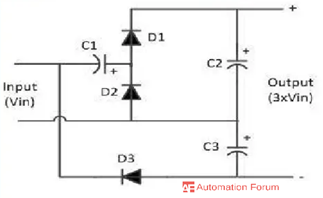# Voltage multiplier and their types

What is a voltage multiplier

Voltage multipliers are used to develop high voltages where low current is needed, it is an arrangement of capacitors and rectifier diodes that are used to generate high voltage DC. Basically it is an electrical circuit that consists of diodes and capacitors, by using the diodes and capacitors voltage is increased and converted from AC to DC. Individual voltage multiplier circuits can be connected in series to obtain even higher output voltages. Just like transformers when the voltage is stepped up the output current decreases for voltage multipliers, although the measured output voltage of a voltage multiplier may be several times greater than the input voltage, once a load is connected the value of output voltage decreases. Also, any small fluctuation of the load impedance causes a large fluctuation in the output voltage of the multiplier. Due to this, they are used only in special applications, such as the load is constant and has high impedance or in the case where the input voltage stability is not critical. Voltage multipliers can increase the voltage by the use of series aiding voltage sources.

How does voltage multiplier works

Most of the multiplier circuits have rectifiers and capacitors the operating principle of the multiplier circuit is mostly the same. Capacitors are charged and discharged during the alternate half cycles of the supplied voltage additional capacitor and rectifier does the voltage increment across the capacitor which is in series the output voltage is the sum of these series capacitor voltages.

What are the types of voltage multiplier

Voltage doubler

The voltage doubler is an electronic voltage multiplier circuit that doubles the voltage by using the charging and discharging principle of capacitors, it consists of capacitors and diodes. The voltage doubler circuit can be simple rectifier circuit that takes the AC voltage as input and generates DC voltage as output and it will be approximate twice the AC voltage.

Half-wave voltage doubler

The working of this voltage doubler the capacitor C1 charges during the negative half-cycle of the input voltage it charges through the rectifier CR1 and it will reach a voltage of VM during the positive half cycle the input voltage will be in series with the voltage of C1. Then capacitor C2 will charge through rectifier CR2 and its voltage level will be 2vm. Capacitor C1 helps the charging of capacitor C2, capacitor C1 sees the alternating current while C2 sees only direct current in this the output voltage and the input signal has the same ripple frequency.

Applications of half-wave voltage doubler

It is used for accelerating purposes in the cathode ray tube of an oscilloscope and it is also used in vacuum tube voltmeters.

Full-wave voltage doubler

During the positive half cycle of the secondary voltage capacitor, C1 charges through D1 to peak voltage of the secondary winding and the capacitor C2 is not charged as the diode D2 is reverse biased. The capacitor C2 charges through D2 to peak voltage of secondary winding when the voltage at point A is negative with respect to point B. but the capacitor C1 cannot discharge as the diode D1 is reverse biased at this instant. The two capacitors are in series and the voltage across the load will be twice the peak secondary voltage, the ripple frequency is twice the line frequency. One of the output capacitors is being charged during each half cycle and this is a full-wave rectifier.

Applications of full-wave voltage doubler

It can be used to provide two DC output voltages of opposite polarity with respect to the input ground.

They have no common connection between the ground of the input and the ground of the output, this disadvantage is not seen in half-wave voltage doubler.

Voltage triplersVoltage tripler is used to increase the voltage coming from an electrical supply. Voltage triplers mostly convert AC to DC, the tripler will increase the input voltage by a factor of three. This device needs power to itself and because of it, they lose some of the voltage as it boosts the power input. Voltage tripler has two main components, first one is a half-wave rectifier that boosts the input voltage by half of the total input and the second one is a voltage doubler, this device takes the total voltage of the input and the voltage added by the half-wave rectifier and doubles it, so the result will be three times that of the input

Applications of voltage triplers

They are mostly used to supply extra power to electronic devices such as printers and copiers